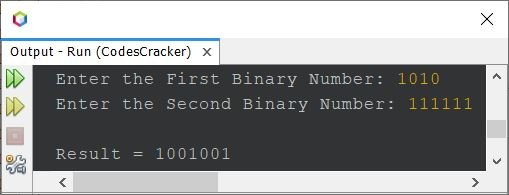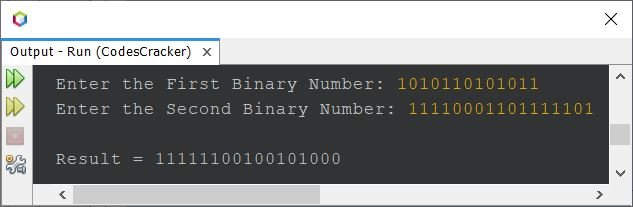# Java Program to Add Two Binary Numbers

This article was created to cover some programs on the addition of two binary numbers in Java. Here, I've created multiple programs for binary number addition in Java.

But before starting the program, let's first remind you how the addition of two binary numbers performs:

```1 + 0 = 1
0 + 1 = 1
0 + 0 = 0
1 + 1 = 10 (0 and carry 1)
1 + 1 + 1 = 10 + 1 = 11 (1 and carry 1)```

For example

```  1 1 1 0 1
+ 1 1 1 1 1
-----------
1 1 1 1 0 0```

## Binary Number Addition in Java Using Integers

The question is: write a Java program to add two binary numbers. Both binary numbers must be entered by the user at runtime. The answer to this question is given below.

The complete version of the binary number addition program in Java was created at the end of this article. But before creating the complete version of the program that performs addition on two binary numbers entered by the user, Let's first create a very basic program that provides easy-to-understand code. This program is created using the int (integer) data type.

```import java.util.Scanner;

public class CodesCracker
{
public static void main(String[] args)
{
int binaryOne, binaryTwo, remOne, remTwo, i=0, carry=0;
int[] res = new int;
Scanner scan = new Scanner(System.in);

System.out.print("Enter the First Binary Number: ");
binaryOne = scan.nextInt();
System.out.print("Enter the Second Binary Number: ");
binaryTwo = scan.nextInt();

while(binaryOne!=0)
{
remOne = binaryOne%10;
remTwo = binaryTwo%10;
if(remOne==0 && remTwo==0)
{
if(carry==0)
{
res[i] = (res[i]*10) + 0;
}
else
{
res[i] = (res[i]*10) + 1;
carry = 0;
}
}
else if(remOne==0 && remTwo==1)
{
if(carry==0)
{
res[i] = (res[i]*10) + 1;
}
else
{
res[i] = (res[i]*10) + 0;
carry = 1;
}
}
else if(remOne==1 && remTwo==0)
{
if(carry==0)
{
res[i] = (res[i]*10) + 1;
}
else
{
res[i] = (res[i]*10) + 0;
carry = 1;
}
}
else if(remOne==1 && remTwo==1)
{
if(carry==0)
{
res[i] = (res[i]*10) + 0;
carry = 1;
}
else
{
res[i] = (res[i]*10) + 1;
carry = 1;
}
}
else
{
System.out.println("\nInvalid Input!");
return;
}
binaryOne = binaryOne/10;
binaryTwo = binaryTwo/10;
i++;
}
if(carry==1)
res[i] = (res[i]*10) + 1;
System.out.print("\nResult = ");
while(i>=0)
{
System.out.print(res[i]);
i--;
}
}
}```

Here is its sample run with user input 11101 as the first and 11111 as the second binary number:The above program has limitations, such as what if the user enters two binary numbers in which one has a greater or lesser number of digits than the other, or what if the user enters a binary number whose size is bigger than int? Therefore, let's modify the above program and recreate the same program using long data types. Also, this program is basically a short version of the previous

```import java.util.Scanner;

public class CodesCracker
{
public static void main(String[] args)
{
long binaryOne, binaryTwo;
int remOne, remTwo, sumDigit, i=0, carry=0;
int[] sum = new int;
Scanner scan = new Scanner(System.in);

System.out.print("Enter the First Binary Number: ");
binaryOne = scan.nextLong();
System.out.print("Enter the Second Binary Number: ");
binaryTwo = scan.nextLong();

while(binaryOne!=0 || binaryTwo!=0)
{
remOne = (int)(binaryOne%10);
remTwo = (int)(binaryTwo%10);
sumDigit = remOne + remTwo + carry;
sum[i] = (int)(sumDigit%2);
carry = (int)(sumDigit/2);
binaryOne = binaryOne/10;
binaryTwo = binaryTwo/10;
i++;
}
if(carry==1)
sum[i] = carry;
System.out.print("\nResult = ");
while(i>=0)
{
System.out.print(sum[i]);
i--;
}
}
}```

Here is its sample run with user input 1010 as the first and 111111 as the second binary number:Let's again shorten the above program. Here is the short version of the above program. The i++ is the post-increment of i, which means the current value of i gets used and then incremented by 1:

```import java.util.Scanner;

public class CodesCracker
{
public static void main(String[] args)
{
long binaryOne, binaryTwo;
int remOne, remTwo, sumDigit, i=0, carry=0;
int[] sum = new int;
Scanner scan = new Scanner(System.in);

System.out.print("Enter Two Binary Numbers: ");
binaryOne = scan.nextLong();
binaryTwo = scan.nextLong();

while(binaryOne!=0 || binaryTwo!=0)
{
sum[i++] = (int)((binaryOne%10 + binaryTwo%10 + carry)%2);
carry = (int)((binaryOne%10 + binaryTwo%10 + carry)/2);
binaryOne /= 10;
binaryTwo /= 10;
}
if(carry==1)
sum[i] = carry;
System.out.print("\nResult = ");
while(i>=0)
System.out.print(sum[i--]);
}
}```

Still, the program is not perfect because I've used an array whose limit is 10. I know you can increase the limit by 100 or more. But what about the data type long, whose limit is 9,223,372,036,854,775,807.

Therefore, I must recommend that you go with the string type to add any two binary numbers without depending on their size or the number of digits the given binary numbers have.

## Binary Number Addition in Java Using Strings

Before creating the complete version, let's first create a simple and basic version using strings. This program assumes that the input of two binary numbers from the user at run-time is of the same number of digits:

```import java.util.Scanner;

public class CodesCracker
{
public static void main(String[] args)
{
String binaryOne, binaryTwo, resRev="", resOrig="";
char charOne, charTwo, carry='0';
int i;

Scanner scan = new Scanner(System.in);

System.out.print("Enter Two Binary Numbers: ");
binaryOne = scan.next();
binaryTwo = scan.next();

for(i=(binaryOne.length()-1); i>=0; i--)
{
charOne = binaryOne.charAt(i);
charTwo = binaryTwo.charAt(i);
if(charOne=='0' && charTwo=='0')
{
if(carry=='0')
resRev = resRev + "0";
else
{
resRev = resRev + "1";
carry = '0';
}
}
else if(charOne=='0' && charTwo=='1')
{
if(carry=='0')
resRev = resRev + "1";
else
{
resRev = resRev + "0";
carry = '1';
}
}
else if(charOne=='1' && charTwo=='0')
{
if(carry=='0')
resRev = resRev + "1";
else
{
resRev = resRev + "0";
carry = '1';
}
}
else if(charOne=='1' && charTwo=='1')
{
if(carry=='0')
{
resRev = resRev + "0";
carry = '1';
}
else
{
resRev = resRev + "1";
carry = '1';
}
}
else
{
System.out.println("\nInvalid Input!");
return;
}
}
if(carry=='1')
resRev = resRev + "1";
for(i=(resRev.length()-1); i>=0; i--)
resOrig = resOrig + resRev.charAt(i);
System.out.println("\nResult = " + resOrig);
}
}```

Here is its sample run with user input 11101 and 11111 as two binary numbers:## Binary Number Addition in Java: Complete Version

Now let's create the complete version of the binary number addition program in Java. I'm calling this program the complete version because it handles all types of errors and also handles binary numbers with any number of digits.

```import java.util.Scanner;

public class CodesCracker
{
public static void main(String[] args)
{
String binaryOne, binaryTwo, resRev="", resOrig="";
char charOne, charTwo, carry='0';
int binaryOneLen, binaryTwoLen, i, j, len;

Scanner scan = new Scanner(System.in);

System.out.print("Enter the First Binary Number: ");
binaryOne = scan.next();
System.out.print("Enter the Second Binary Number: ");
binaryTwo = scan.next();

binaryOneLen = binaryOne.length();
binaryTwoLen = binaryTwo.length();

if(binaryOneLen > binaryTwoLen)
{
for(i=(binaryOneLen-1), j=(binaryTwoLen-1); j>=0; i--, j--)
{
charOne = binaryOne.charAt(i);
charTwo = binaryTwo.charAt(j);
if(charOne=='0' && charTwo=='0')
{
if(carry=='0')
resRev = resRev + "0";
else
{
resRev = resRev + "1";
carry = '0';
}
}
else if(charOne=='0' && charTwo=='1')
{
if(carry=='0')
resRev = resRev + "1";
else
{
resRev = resRev + "0";
carry = '1';
}
}
else if(charOne=='1' && charTwo=='0')
{
if(carry=='0')
resRev = resRev + "1";
else
{
resRev = resRev + "0";
carry = '1';
}
}
else if(charOne=='1' && charTwo=='1')
{
if(carry=='0')
{
resRev = resRev + "0";
carry = '1';
}
else
{
resRev = resRev + "1";
carry = '1';
}
}
else
{
System.out.println("\nInvalid Input!");
return;
}
}
for(i=i; i>=0; i--)
{
charOne = binaryOne.charAt(i);
if(carry=='1')
{
if(charOne=='1')
{
resRev = resRev + "0";
carry = '1';
}
else if(charOne=='0')
{
resRev = resRev + "1";
carry = '0';
}
else
{
System.out.println("\nInvalid Input!");
return;
}
}
else
{
resRev = resRev + charOne;
}
}
}
else if(binaryOneLen < binaryTwoLen)
{
for(i=(binaryOneLen-1), j=(binaryTwoLen-1); i>=0; i--, j--)
{
charOne = binaryOne.charAt(i);
charTwo = binaryTwo.charAt(j);
if(charOne=='0' && charTwo=='0')
{
if(carry=='0')
resRev = resRev + "0";
else
{
resRev = resRev + "1";
carry = '0';
}
}
else if(charOne=='0' && charTwo=='1')
{
if(carry=='0')
resRev = resRev + "1";
else
{
resRev = resRev + "0";
carry = '1';
}
}
else if(charOne=='1' && charTwo=='0')
{
if(carry=='0')
resRev = resRev + "1";
else
{
resRev = resRev + "0";
carry = '1';
}
}
else if(charOne=='1' && charTwo=='1')
{
if(carry=='0')
{
resRev = resRev + "0";
carry = '1';
}
else
{
resRev = resRev + "1";
carry = '1';
}
}
else
{
System.out.println("\nInvalid Input!");
return;
}
}
for(i=j; i>=0; i--)
{
charTwo = binaryTwo.charAt(i);
if(carry=='1')
{
if(charTwo=='1')
{
resRev = resRev + "0";
carry = '1';
}
else if(charTwo=='0')
{
resRev = resRev + "1";
carry = '0';
}
else
{
System.out.println("\nInvalid Input!");
return;
}
}
else
{
resRev = resRev + charTwo;
}
}
}
else
{
for(i=(binaryOneLen-1); i>=0; i--)
{
charOne = binaryOne.charAt(i);
charTwo = binaryTwo.charAt(i);
if(charOne=='0' && charTwo=='0')
{
if(carry=='0')
resRev = resRev + "0";
else
resRev = resRev + "1";
}
else if(charOne=='0' && charTwo=='1')
{
if(carry=='0')
resRev = resRev + "1";
else
{
resRev = resRev + "0";
carry = '1';
}
}
else if(charOne=='1' && charTwo=='0')
{
if(carry=='0')
resRev = resRev + "1";
else
{
resRev = resRev + "0";
carry = '1';
}
}
else if(charOne=='1' && charTwo=='1')
{
if(carry=='0')
{
resRev = resRev + "0";
carry = '1';
}
else
{
resRev = resRev + "1";
carry = '1';
}
}
else
{
System.out.println("\nInvalid Input!");
return;
}
}
}
if(carry=='1')
resRev = resRev + "1";
len = resRev.length();
for(i=len-1; i>=0; i--)
resOrig = resOrig + resRev.charAt(i);
System.out.println("\nResult = " + resOrig);
}
}```

Here is its sample run with user input 1010110101011 as the first and 11110001101111101 as the second number:I know the program is a little longer, but I recommend you take a deep breath to understand the logic used in the program. There are many other ways, too, to create the same program. Since the article is getting longer than usual, I'm letting you modify the program and create another way for yourself.

Java Online Test

« Previous Program Next Program »